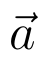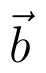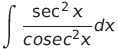Select Page

# MCQ Solutions for CBSE 12 Science Maths Vector Algebra in English

MCQ Solutions for CBSE 12 Science Maths Vector Algebra in English to enable students to get Solutions in a narrative video format for the specific question.

Expert Teacher provides MCQ Solutions for CBSE 12 Science Maths Vector Algebra through Video Solutions in English language. This video solution will be useful for students to understand how to write an answer in exam in order to score more marks. This teacher uses a narrative style for a question from Vector Algebra not only to explain the proper method of answering question, but deriving right answer too.

Please find the question below and view the Solution in a narrative video format.

Question:

Solution Video in English:

You can select video Solutions from other languages also. Please check Solutions in ( Hindi )

## Similar Questions from CBSE, 12th Science, Maths, Vector Algebra

Question 1 : if a, b, c are unit vectors such that a + b + c = 0, then find the value of a.b + b.c + c.a. (View Answer Video)

Question 2 :  Ifanddenote the position vectors of points A and B respectively and C is a point on AB such that AC = 2 CB, then write the position vector of C.  (View Answer Video)

Question 3 : Find the projection of the vectoron the vector(View Answer Video)

Question 4 : Find |x|, if for a unit vector a,(x - a).(x + a) = 12. (View Answer Video)

Question 5 : Find a unit vector parallel to the sum of vectorsand(View Answer Video)

### Continuity and Differentiability

Question 1 : Differentiate w.r.t.x the function, for some fixed a > 0 and x > 0. (View Answer Video)

Question 2 : Differentiatew.r.t.x. (View Answer Video)

Question 3 : Findfor the function. (View Answer Video)

Question 4 : If, findin terms of y alone. (View Answer Video)

Question 5 : Findfor the function. (View Answer Video)

### Integrals

Question 1 : Evaluate :(View Answer Video)

Question 2 : Write the value of:(View Answer Video)

Question 3 :(View Answer Video)

Question 4 : Find :(View Answer Video)

Question 5 : Find. (View Answer Video)

### Determinant

Question 1 : If A is a symmetric matrix, then? (View Answer Video)

Question 2 : If A is an invertible matrix of order 2, thenis equal to, (View Answer Video)

Question 3 : The following system of equations has x + 3y + 3z = 2, x + 4y + 3z = 1, x + 3y + 4z = 2,

Question 4 : Let, where. THhen (View Answer Video)